Creating and training convolutional neural networks¶

We will now improve upon our previous example by creating some more sophisticed image classifiers and using a more challanging dataset. Specifically, we will implement convolutional neural networks (CNNs) and train them using the CIFAR10 dataset, which uses natural color images. This dataset uses 60000 small color images of size 32x32x3 (the 3 is for the RGB color channels) and 10 class labels. 50000 of these are used for training and the remaining 10000 are for the test set. There is also a CIFAR100 version that uses 100 class labels, but we will only use CIFAR10 here.

airplane automobile bird cat deer dog frog horse ship truck:

# Install Chainer and CuPy!

!curl https://colab.chainer.org/install | sh -

Reading package lists... Done
Building dependency tree
0 upgraded, 0 newly installed, 0 to remove and 1 not upgraded.
Requirement already satisfied: fastrlock>=0.3 in /usr/local/lib/python3.6/dist-packages (from cupy-cuda80==4.0.0b3)
Requirement already satisfied: numpy>=1.9.0 in /usr/local/lib/python3.6/dist-packages (from cupy-cuda80==4.0.0b3)
Requirement already satisfied: six>=1.9.0 in /usr/local/lib/python3.6/dist-packages (from cupy-cuda80==4.0.0b3)
Requirement already satisfied: chainer==4.0.0b3 in /usr/local/lib/python3.6/dist-packages
Requirement already satisfied: numpy>=1.9.0 in /usr/local/lib/python3.6/dist-packages (from chainer==4.0.0b3)
Requirement already satisfied: filelock in /usr/local/lib/python3.6/dist-packages (from chainer==4.0.0b3)
Requirement already satisfied: six>=1.9.0 in /usr/local/lib/python3.6/dist-packages (from chainer==4.0.0b3)
Requirement already satisfied: protobuf>=3.0.0 in /usr/local/lib/python3.6/dist-packages (from chainer==4.0.0b3)
Requirement already satisfied: setuptools in /usr/lib/python3/dist-packages (from protobuf>=3.0.0->chainer==4.0.0b3)


1. Define the Model¶

As in the previous examples, we define our model as a subclass of Chain. Our CNN model will have three layers of convolutions followed by two fully connected layers. Although this is still a fairly small CNN, it will still significantly outperform a fully-connected model. After completing this notebook, you are encouraged to try an experiment yourself to verify this, such as by using the MLP from the previous example or similar.

Recall from the first hands-on example that we define a model as follows.

1. Inside the initializer of the model chain class, provide the names and corresponding layer objects as keyword arguments to parent(super) class.
2. Define a __call__ method so that we can call the chain like a function. This method is used to implement the forward computation.
:

import chainer
import chainer.functions as F

class MyModel(chainer.Chain):

def __init__(self, n_out):
super(MyModel, self).__init__()
with self.init_scope():
self.conv1=L.Convolution2D(None, 32, 3, 3, 1)
self.conv2=L.Convolution2D(32, 64, 3, 3, 1)
self.conv3=L.Convolution2D(64, 128, 3, 3, 1)
self.fc4=L.Linear(None, 1000)
self.fc5=L.Linear(1000, n_out)

def __call__(self, x):
h = F.relu(self.conv1(x))
h = F.relu(self.conv2(h))
h = F.relu(self.conv3(h))
h = F.relu(self.fc4(h))
h = self.fc5(h)
return h

/usr/local/lib/python3.6/dist-packages/cupy/core/fusion.py:659: FutureWarning: cupy.core.fusion is experimental. The interface can change in the future.
util.experimental('cupy.core.fusion')


2. Train the model¶

Let’s define a ‘train’ function that we can also use to train other models easily later on. This function takes a model object, trains the model to classify the 10 CIFAR10 classes, and finally returns the trained model.

We will use this train function to train the MyModel network defined above.

:

from chainer.datasets import cifar
from chainer import iterators
from chainer import optimizers
from chainer import training
from chainer.training import extensions

def train(model_object, batchsize=64, gpu_id=0, max_epoch=20):

# 1. Dataset
train, test = cifar.get_cifar10()

# 2. Iterator
train_iter = iterators.SerialIterator(train, batchsize)
test_iter = iterators.SerialIterator(test, batchsize, False, False)

# 3. Model
model = L.Classifier(model_object)
if gpu_id >=0:
model.to_gpu(gpu_id)

# 4. Optimizer
optimizer.setup(model)

# 5. Updater
updater = training.StandardUpdater(train_iter, optimizer, device=gpu_id)

# 6. Trainer
trainer = training.Trainer(updater, (max_epoch, 'epoch'), out='{}_cifar10_result'.format(model_object.__class__.__name__))

# 7. Evaluator

class TestModeEvaluator(extensions.Evaluator):

def evaluate(self):
model = self.get_target('main')
ret = super(TestModeEvaluator, self).evaluate()
return ret

trainer.extend(extensions.LogReport())
trainer.extend(TestModeEvaluator(test_iter, model, device=gpu_id))
trainer.extend(extensions.PrintReport(['epoch', 'main/loss', 'main/accuracy', 'validation/main/loss', 'validation/main/accuracy', 'elapsed_time']))
trainer.extend(extensions.PlotReport(['main/loss', 'validation/main/loss'], x_key='epoch', file_name='loss.png'))
trainer.extend(extensions.PlotReport(['main/accuracy', 'validation/main/accuracy'], x_key='epoch', file_name='accuracy.png'))
trainer.run()
del trainer

return model

gpu_id = 0  # Set to -1 if you don't have a GPU

model = train(MyModel(10), gpu_id=gpu_id)

Downloading from https://www.cs.toronto.edu/~kriz/cifar-10-python.tar.gz...

epoch       main/loss   main/accuracy  validation/main/loss  validation/main/accuracy  elapsed_time
1           1.52065     0.445912       1.30575               0.527568                  9.28
2           1.22656     0.559399       1.20226               0.571059                  18.0121
3           1.07178     0.616637       1.14007               0.593451                  26.0337
4           0.954909    0.658791       1.09014               0.617237                  34.1482
5           0.849026    0.696152       1.05939               0.633161                  42.2791
6           0.748826    0.732514       1.05501               0.64172                   50.343
7           0.645085    0.769486       1.07674               0.63963                   58.4476
8           0.542578    0.806218       1.13905               0.643909                  66.4593
9           0.438032    0.845169       1.14717               0.648985                  74.6315
10          0.351087    0.8762         1.27986               0.642118                  82.7441
11          0.268679    0.90741        1.41233               0.63545                   91.8396
12          0.201693    0.931978       1.55193               0.635549                  99.9606
13          0.163686    0.944393       1.7719                0.64172                   108.078
14          0.137398    0.952665       1.89784               0.635947                  116.18
15          0.120794    0.958527       2.02123               0.636943                  124.336
16          0.105321    0.964309       2.09981               0.631668                  132.463
17          0.0979085   0.966073       2.23514               0.632564                  140.565
18          0.10191     0.965389       2.2047                0.629678                  148.612
19          0.0889873   0.96937        2.40244               0.623806                  156.785
20          0.0798767   0.973191       2.4482                0.630175                  165.914


The training has completed. Let’s take a look at the results.

:

from IPython.display import Image
Image(filename='MyModel_cifar10_result/loss.png')

: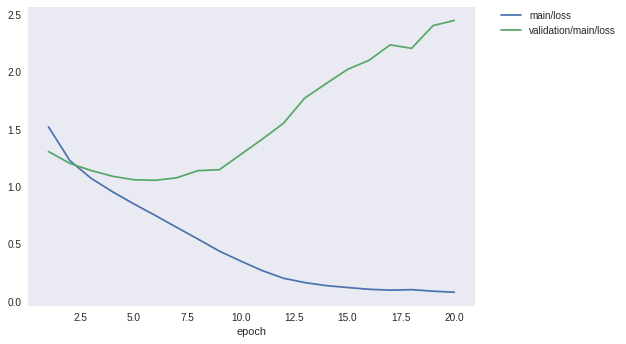:

Image(filename='MyModel_cifar10_result/accuracy.png')

: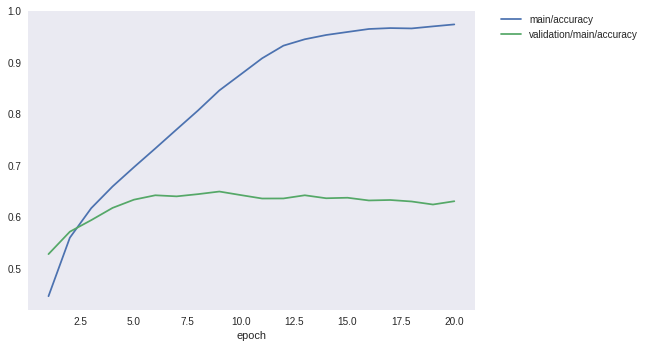Although the accuracy on the training set reach 98%, the loss on the test set started increasing after 5 epochs and the test accuracy plateaued round 60%. It looks like the model is overfitting to the training data.

3. Prediction using our trained model¶

Although the test accuracy is only around 60%, let’s try to classify some test images with this model.

:

%matplotlib inline
import matplotlib.pyplot as plt

cls_names = ['airplane', 'automobile', 'bird', 'cat', 'deer',
'dog', 'frog', 'horse', 'ship', 'truck']

def predict(model, image_id):
_, test = cifar.get_cifar10()
x, t = test[image_id]
model.to_cpu()
y = model.predictor(x[None, ...]).data.argmax(axis=1)
print('predicted_label:', cls_names[y])

plt.imshow(x.transpose(1, 2, 0))
plt.show()

for i in range(5):
predict(model, i)

predicted_label: dog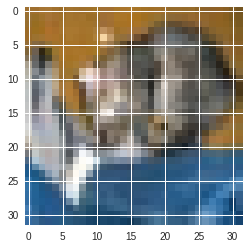predicted_label: ship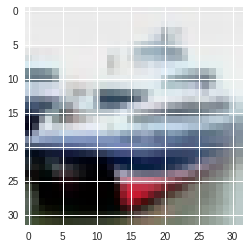predicted_label: ship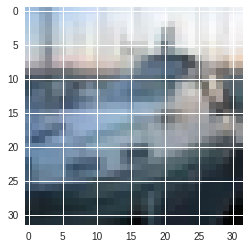predicted_label: airplane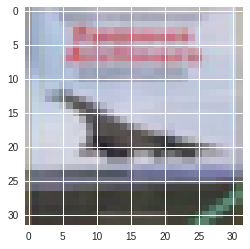predicted_label: deer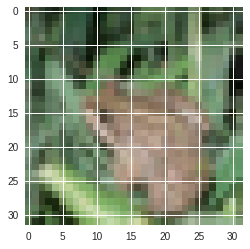Some are correctly classified, others are not. Even though the model can predict the classification using the training datase with 100% accuracy, it is meaningless if we cannot generalize to (previously unseen) test data. The accuracy of on the test set data is believed to estimate generalization ability more directly.

How can we design and train a model with better generalization ability?

4. Create a deeper model¶

Let’s try making our CNN deeper by adding more layers and see how it performs. We will also make our model modular by writing it as the combination of three chains. This will help to improve readability and reduce code duplication: - A single convolutional neural net, ConvBlock - A single fully connected neural net, LinearBlock - Create a full model by chaining many of these two blocks together

Define the block of layers¶

Let’s define the network blocks, ConvBlock and LinearBlock, which will be stacked to create the full model.

[ ]:

class ConvBlock(chainer.Chain):

def __init__(self, n_ch, pool_drop=False):
w = chainer.initializers.HeNormal()
super(ConvBlock, self).__init__()
with self.init_scope():
self.conv = L.Convolution2D(None, n_ch, 3, 1, 1,
nobias=True, initialW=w)
self.bn = L.BatchNormalization(n_ch)

self.pool_drop = pool_drop

def __call__(self, x):
h = F.relu(self.bn(self.conv(x)))
if self.pool_drop:
h = F.max_pooling_2d(h, 2, 2)
h = F.dropout(h, ratio=0.25)
return h

class LinearBlock(chainer.Chain):

def __init__(self):
w = chainer.initializers.HeNormal()
super(LinearBlock, self).__init__()
with self.init_scope():
self.fc = L.Linear(None, 1024, initialW=w)

def __call__(self, x):
return F.dropout(F.relu(self.fc(x)), ratio=0.5)


ConvBlock is defined by inheriting Chain. It contains a single convolution layer and a Batch Normalization layer registered by the constructor. The __call__ method recieves the data and applies activation funtion to it. If pool_drop is set to True, the Max_Pooling and Dropout functions are also applied.

Let’s now define the deeper CNN network by stacking the component blocks.

[ ]:

class DeepCNN(chainer.ChainList):

def __init__(self, n_output):
super(DeepCNN, self).__init__(
ConvBlock(64),
ConvBlock(64, True),
ConvBlock(128),
ConvBlock(128, True),
ConvBlock(256),
ConvBlock(256, True),
LinearBlock(),
LinearBlock(),
L.Linear(None, n_output)
)

def __call__(self, x):
for f in self.children():
x = f(x)
return x


Note that DeepCNN inherits from ChainList instead of Chain. The ChainList class inherits from Chain and is very useful when you define networks that consist of a long sequence of Link and/or Chain layers.

Note also the difference in the way that links and/or chains are supplied to the initializer of ChainList; they are passed as normal arguments, not as keyword arguments. Also, in the __call__ method, they are retreived from the list in the order they were registered by calling the self.children() method.

This feature enables us to describe the forward propagation very concisely. With the component list returned by self.children(), we can write the entire forward network by using a for loop to access each component chain one after another. Then we can first set the input ‘x’ to the first net and its output is passed to the next series of ‘Link’ or ‘Chain’.

:

gpu_id = 0  # Set to -1 if you don't have a GPU

model = train(DeepCNN(10), gpu_id=gpu_id)

epoch       main/loss   main/accuracy  validation/main/loss  validation/main/accuracy  elapsed_time
1           1.97534     0.284127       1.52095               0.429638                  51.7811
2           1.47785     0.447223       1.30206               0.584594                  136.648
3           1.23227     0.553377       1.06446               0.660032                  240.008
4           1.04628     0.630702       0.964976              0.695163                  343.388
5           0.902084    0.685642       0.912458              0.695561                  447.047
6           0.776821    0.729373       0.744387              0.764132                  550.3
7           0.683545    0.768286       0.631135              0.798069                  653.239
8           0.598311    0.798315       0.593679              0.804339                  756.449
9           0.53423     0.822011       0.60011               0.80623                   859.992
10          0.482092    0.837708       0.502585              0.832803                  963.425
11          0.42906     0.855994       0.446699              0.851811                  1066.43
12          0.389187    0.869638       0.431314              0.862261                  1169.77
13          0.357603    0.879436       0.431607              0.857484                  1273.36
14          0.326755    0.889165       0.433513              0.862162                  1376.66
15          0.300896    0.899248       0.555515              0.814192                  1479.75
16          0.278662    0.90739        0.439382              0.864351                  1582.91
17          0.250386    0.914242       0.470831              0.861266                  1685.86
18          0.235094    0.921875       0.464271              0.865346                  1788.89
19          0.228264    0.923716       0.429198              0.872313                  1891.77
20          0.20953     0.930038       0.448946              0.865545                  1994.67


The training is completed. Let’s take a look at the loss and accuracy.

:

Image(filename='DeepCNN_cifar10_result/loss.png')

: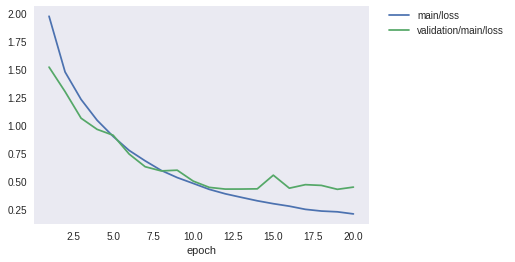:

Image(filename='DeepCNN_cifar10_result/accuracy.png')

: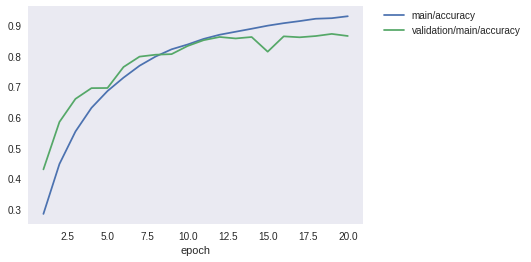Now the accuracy on the test set has improved a lot compared to the previous smaller CNN. Previously the accuracy was around 60% and now it is around 87%. According to current research reports, the most advanced model can reach around 97%. To improve the accuracy more, it is necessary not only to improve the models but also to increase the training data (Data augmentation) or to combine multiple models to carry out the best perfomance (Ensemble method). You may also find it interesting to experiment with some larger and more difficult datasets. There is more room for improvement by your new ideas!